# Home Loans: How To Compute The Effective And Nominal Interest Rates?

by • April 19, 2013 • Residential Property LoanComments (4)44046

# Home Loans: How To Compute The Effective And Nominal Interest Rates?

Interest rate is the cost of borrowing. But the interest rate stated in our credit cards or loans applications may not be the true cost. The rate given is the per annum (p.a.) rate. For example, in a home loan letter of offer you may find a line that says, “First year interest rate is 1% p.a.”. But since the base period for interest calculation (compounding or conversion) can be more than once a year, there can be a difference in interest cost for loans with the same interest rate per annum, loan amount and duration.

The frequency in which the outstanding loan amount is calculated, is also known as rest.

Most Singapore home loans follow a monthly rest, while a few is on daily- and annual-rest basis. All else being equal (including the frequency of instalments), a shorter rest will reduce interest payment.

Thus an effective annual interest rate is needed to measure the true borrowing cost. The interest rate per annum is only the nominal interest rate. This nominal rate is equal to the effective rate when a loan is on annual-rest basis and instalments are also made annually.

In the reminder of this article, I will attempt to teach you how to obtain the annual effective rate and nominal rate, with formulas or Excel, for loans with different frequencies of compounding.

## What is the maths formula for the annual effective rate?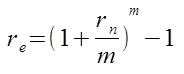—— Eq 1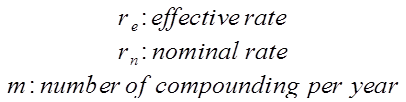The annual effective interest rate, re, is actually the ratio of the amount of interest earned during a year to the amount of principal outstanding at the beginning of the year.

The above equation, Eq 1, shows the relation between the effective rate and nominal rate.

So, if you take a monthly-rest loan with an interest rate of 5% p.a., to find the annual effective rate you first define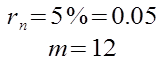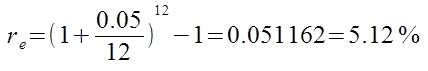An important point to note is that the effective rate is always larger than the nominal if compounding is done more than once a year, m > 1.

For a daily compounding loan, the
m = 365, and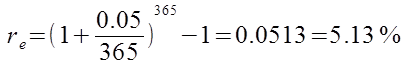Table 1 presents the effective rate across the three different rests:

Table 1

 Type of loan Effective rate (%) Daily-rest with daily instalments 5.13 Monthly-rest with monthly instalments 5.12 Annual-rest with annual instalments 5.00

Please note that the effective interest is lower (reduced interest payment) for shorter rest loans only if the frequency of instalments are the same, and all else are equal. For example, comparing a daily-rest loan with monthly instalments and a monthly-rest loan with monthly instalments, the former will have a lower effective rate.

## What is the maths formula for the annual nominal rate?

Conversely, if you have the effective rate, but wish to find the nominal. You can use the below formula: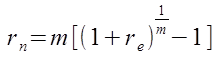—– Eq 2

Using the same example, and inserting the values into Eq 2: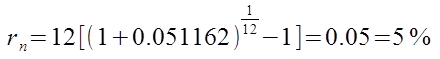we obtain the nominal rate of 5%, as expected.

## What are the Excel functions?

Instead of doing the computation with a calculator, you can let Excel do it for you.

Table 2: Maths Formulas and Excel Functions

 Maths Formula Excel Function= EFFECT (nominal_rate, npery)= NOMINAL (effect_rate, npery)

where npery is equivalent to m, or the number of compounding per year.

But before you can uitlise these two Excel functions, you need to include an add-on – the Excel Analysis ToolPak. To do so in Excel 2007, you have to click on the Office button on the top left- hand corner as in Figure 1.

Figure 1Next, you click on Excel Options, and a window will pop up as in Figure 2.

Figure 2Select Add-Ins, and this will bring you to the next screen:

Figure 3Under “Manage”, make sure “Excel Add-ins” is selected than click Go… The Add-Ins window will appear as seen in Figure 4.

Figure 4Mark the check-box for “Analysis ToolPak” and click OK. Now you are all set to use the EFFECT and NOMINAL functions.

For advice on a new home loan.